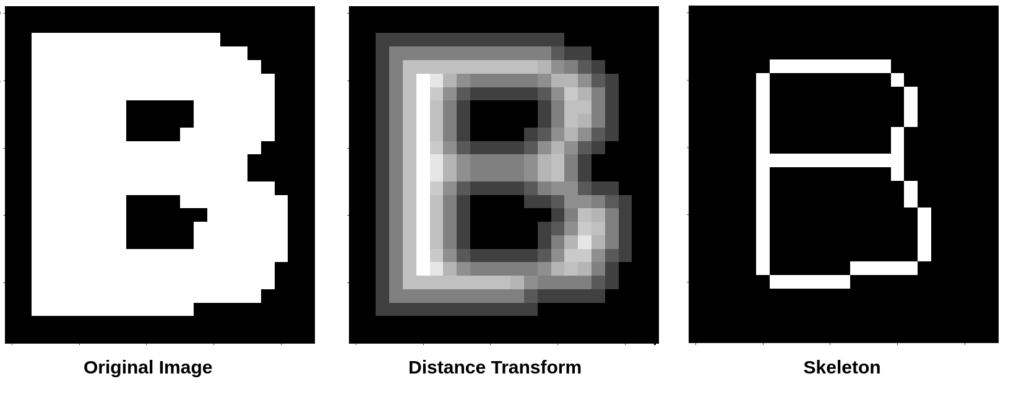# Stroke width identification algorithm for text like regions

In character recognition, the distance between two edges of a stroke, measured perpendicular to the stroke centerline is called the stroke width. Take a look at the below image. The length of lines marked in Red gives the stroke width of the character.

There are quite a few ways to identify the stroke width, In this article I will talk about the one based on distance transform. Distance transform when applied to a binary image gives us an image where each pixel represents it’s distance from the nearest 0 value pixel.

As you can visually calculate, the stroke width in case of the above image is 6. The value at the center in the DT image is 3 that’s because the pixel is 3 units away from the closest 0 value pixel. So we can conclude that given a binary image, the stroke width is the mean of center pixels multipied by 2. And now to find the center pixels we can skeletonize the image which will give use a boolean mask with center pixles marked as True.

Once we have boolean mask, we can easily pick the values of center pixels from the DT image. Python code that does all of this is given below:

``````from skimage.io import imread
from skimage.color import rgb2gray
from skimage.filters import threshold_otsu
from scipy.ndimage.morphology import distance_transform_edt
from skimage.morphology import skeletonize
import numpy as np

# convert the image to grayscale
gray = rgb2gray(img)

# thresholding the image to binary
threshold = threshold_otsu(gray)
binary_image = gray > threshold

# avoid wrong distance calculations for corner pixels

# find the distance transform

# skeletonize the image to find the center pixels

# find the center pixel distances
center_pixel_distances = distances[skeleton]

# get the stroke width, twice the average of center pixel distances
stroke_width = np.mean(center_pixel_distances) * 2

print(stroke_width)
# show the image
plt.figure(figsize=(10,10))

References:

https://homepages.inf.ed.ac.uk/rbf/HIPR2/distance.htm

5 1 vote
Article Rating
Subscribe
Notify of